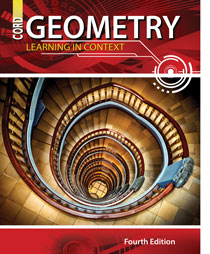# Geometry - 4th Edition

## Chapter 12: Probability

Some links are repeated for use with more than one lesson.

### 12.1 Probability

http://www.mathsisfun.com/data/probability.html
NEW This page defines and gives examples and practice problems for important terms and concepts regarding probability.

http://www.regentsprep.org/regents/math/algebra/apr5/theoprop.htm

### 12.2 The Addition Principle of Counting

http://www.regentsprep.org/Regents/math/algtrig/ATS6/LTheo.htm
NEW This page summarizes the probability calculations for AND and OR of events, including some practice problems.

http://www.mathsteacher.com.au/year10/ch05_probability/05_mutually_exclusive/mut.htm

### 12.3 Independent and Dependent Events

http://www.regentsprep.org/regents/math/algebra/apr6/indexAPR6.htm
NEW This site has several examples and practice problems dealing with events, including independent and dependent events.

http://www.mathsisfun.com/data/probability-events-types.html

### 12.4 Compound Events

http://www.mathsisfun.com/data/probability-events-conditional.html
NEW This page discusses the probability of compound events—when one event is dependent on another event, including practice problems.

http://www.regentsprep.org/regents/math/algebra/apr3/indexAPR3.htm
NEW This site includes links to discussion and practice problems dealing with conditional probability.

### 12.5 Permutations

http://www.regentsprep.org/regents/math/algebra/apr2/indexAPR2.htm
NEW This site deals with the notation and advanced probability calculations using permutations to help determine the sample space.

http://www.mathsisfun.com/combinatorics/combinations-permutations.html
NEW The page discusses and compares the concepts of permutations and combinations (next lesson in your text), including practice problems.

http://www.mathsisfun.com/numbers/factorial.html
NEW This page is a nice presentation of and listing of the first several values for the factorial function, used extensively in probability calculations involving permutations and combinations.

### 12.6 Combinations

http://www.mathsisfun.com/combinatorics/combinations-permutations-calculator.html
NEW A handy permutations and combinations calculator, including the ability to include specific requirements on the resulting arrangements.

http://www.regentsprep.org/Regents/math/algtrig/ATS5/indexATS5.htm
NEW This site includes lessons and practice problems about the notation used in permutations and combinations situations.

### 12.7 Making Decisions Based on Probability

http://www.mathsisfun.com/data/spinner.php
NEW A real-time spinner that can be configured as you like.

http://www.mathsisfun.com/data/survey-conducting.html
NEW A simple guide with tips for conducting surveys to collect sample data.

http://www.baseball-reference.com/teams/
NEW Huge repository of American baseball statistics for all teams and players, useful for numerous probability predictions about the next game!

http://www.nfl.com/stats/player
NEW A similarly huge repository of American football statistics for all teams and players, useful for numerous probability predictions about the next game!

http://www.worldometers.info/
NEW Real-time information from around the world, can be a useful starting point for making probability calculations and statements.